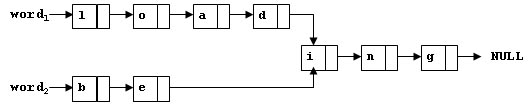# pat a1032 Sharing ------共有后缀

To store English words, one method is to use linked lists and store a word letter by letter. To save some space, we may let the words share the same sublist if they share the same suffix. For example, loading and being are stored as showed in Figure 1.Figure 1

You are supposed to find the starting position of the common suffix (e.g. the position of i in Figure 1).

### Input Specification:

Each input file contains one test case. For each case, the first line contains two addresses of nodes and a positive N (≤10​5​​), where the two addresses are the addresses of the first nodes of the two words, and N is the total number of nodes. The address of a node is a 5-digit positive integer, and NULL is represented by −1.

Then N lines follow, each describes a node in the format:

Address Data Next


whereAddress is the position of the node, Data is the letter contained by this node which is an English letter chosen from { a-z, A-Z }, and Next is the position of the next node.

### Output Specification:

For each case, simply output the 5-digit starting position of the common suffix. If the two words have no common suffix, output -1 instead.

### Sample Input 1:

11111 22222 9
67890 i 00002
00010 a 12345
00003 g -1
12345 D 67890
00002 n 00003
22222 B 23456
11111 L 00001
23456 e 67890
00001 o 00010


### Sample Output 1:

67890


### Sample Input 2:

00001 00002 4
00001 a 10001
10001 s -1
00002 a 10002
10002 t -1


### Sample Output 2:

-1

#include<cstdio>
#include<iostream>
#include<cstring>
using namespace std;

const int maxn=100010;

struct Node
{
char data;
int next;
bool flag;
}node[maxn];

int main()
{
//存储空间的所有节点flag位置为false
for(int i=0;i<maxn;i++)
{
node[i].flag=false;
}
int s1,s2,n;
scanf("%d%d%d",&s1,&s2,&n);

char data;

//n个节点信息的输入
for(int i=0;i<n;i++)
{
}

int p;
//第一条链表的所有节点的flag位置为true
for(p=s1;p!=-1;p=node[p].next)
{
node[p].flag=true;
}

//遍历第二条链表的全部节点，看其中是否有标志位为true的节点
for(p=s2;p!=-1;p=node[p].next)
{
if(node[p].flag==true) break;
}

if(p!=-1)
{
printf("%05d\n",p);
}
else
{
printf("-1\n",p);
}
return 0;
}

©️2019 CSDN 皮肤主题: 技术黑板 设计师: CSDN官方博客